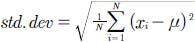NextPrevious

# What is standard deviation?

One common way of reporting the error in a measurement is to report the standard deviation for the set of measurements. Standard deviation is calculated from the formula:where N is the number of trials, xi is the value of the measurement of the ith trial, and μ is the arithmetic mean value of the N measurements.

Close

This is a web preview of the "The Handy Chemistry Answer Book" app. Many features only work on your mobile device. If you like what you see, we hope you will consider buying. Get the App